# Selina Solutions Concise Maths Class 7 Chapter 20: Mensuration (Perimeter and Area of Plane Figures) Exercise 20B

Selina Solutions Concise Maths Class 7 Chapter 20 Mensuration (Perimeter and Area of Plane Figures) Exercise 20B are designed by the experts at BYJU’S to boost confidence among students. The important steps to be followed in finding the area of rectangle, square and triangle are explained in brief under this exercise. The solutions contain explanations in an interactive manner to make learning fun for the students. To gain a better hold on these concepts, students can refer to Selina Solutions Concise Maths Class 7 Chapter 20 Mensuration (Perimeter and Area of Plane Figures) Exercise 20B PDF, from the links which are available below.

## Selina Solutions Concise Maths Class 7 Chapter 20: Mensuration (Perimeter and Area of Plane Figures) Exercise 20B Download PDF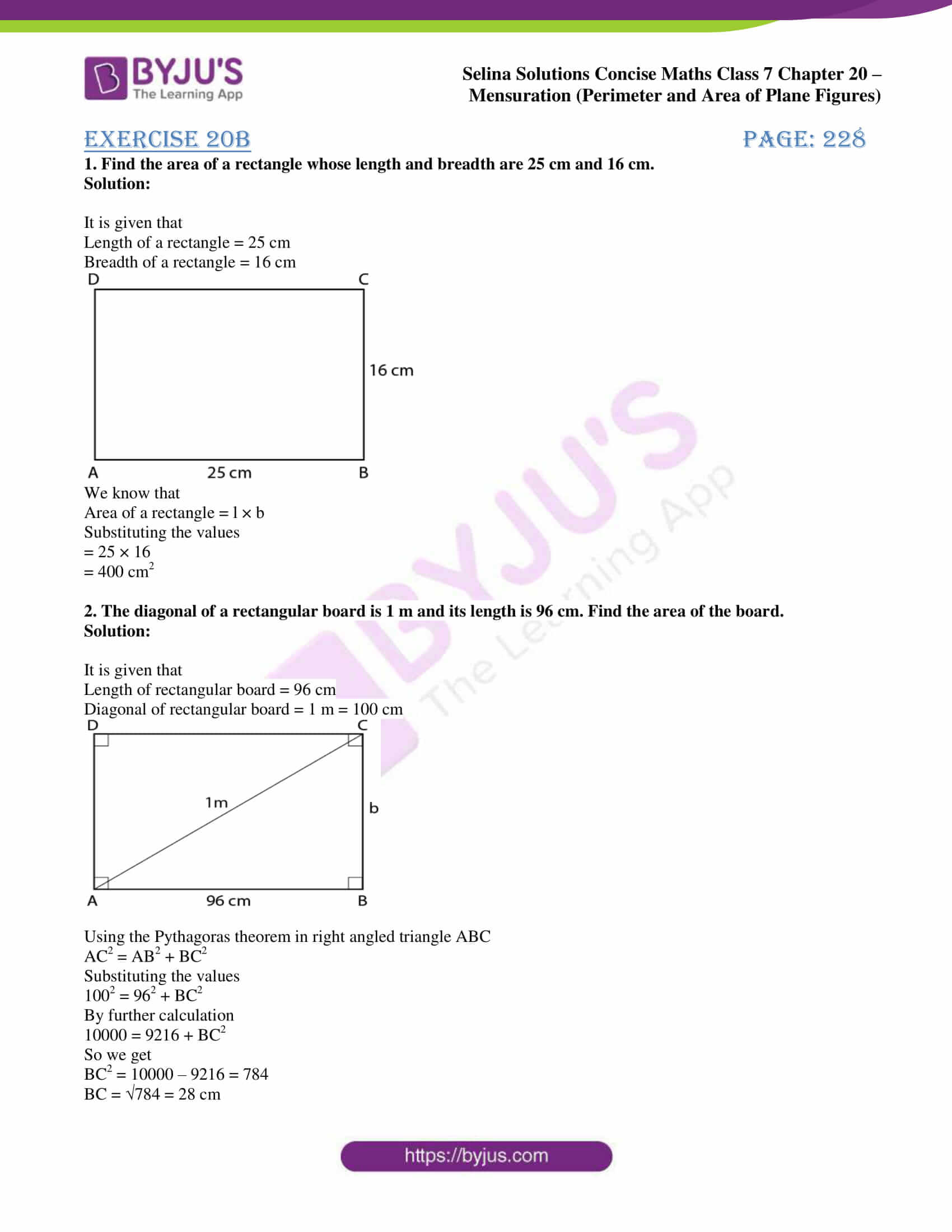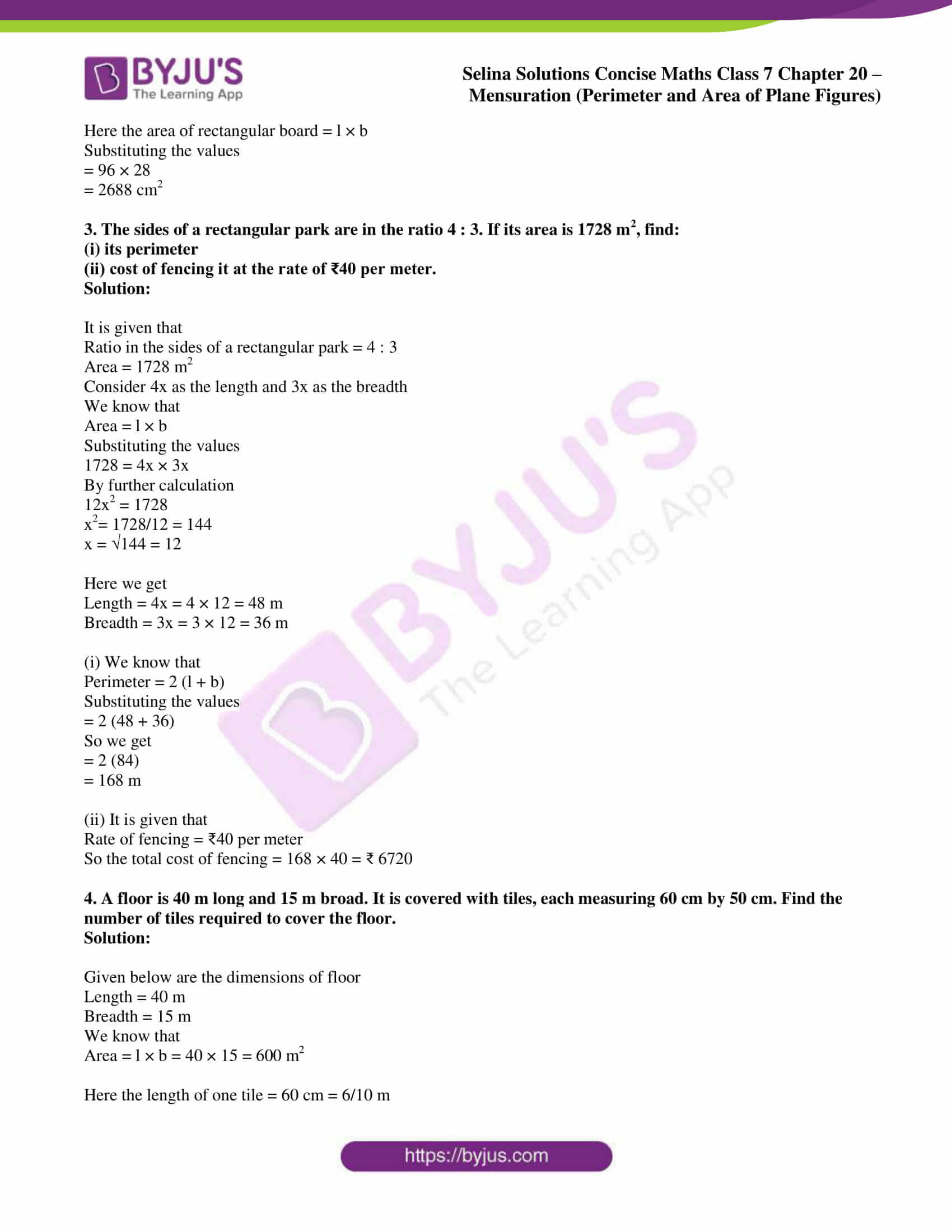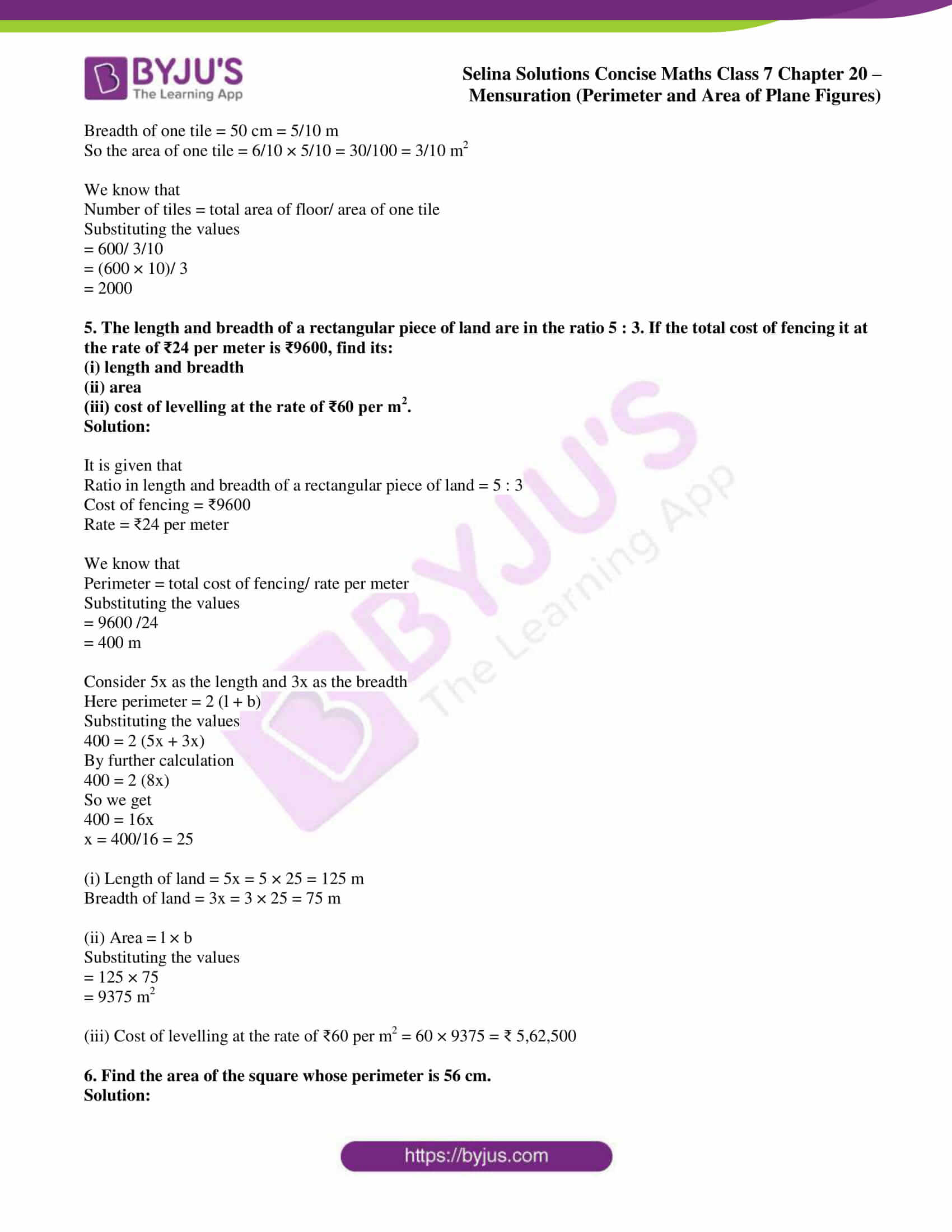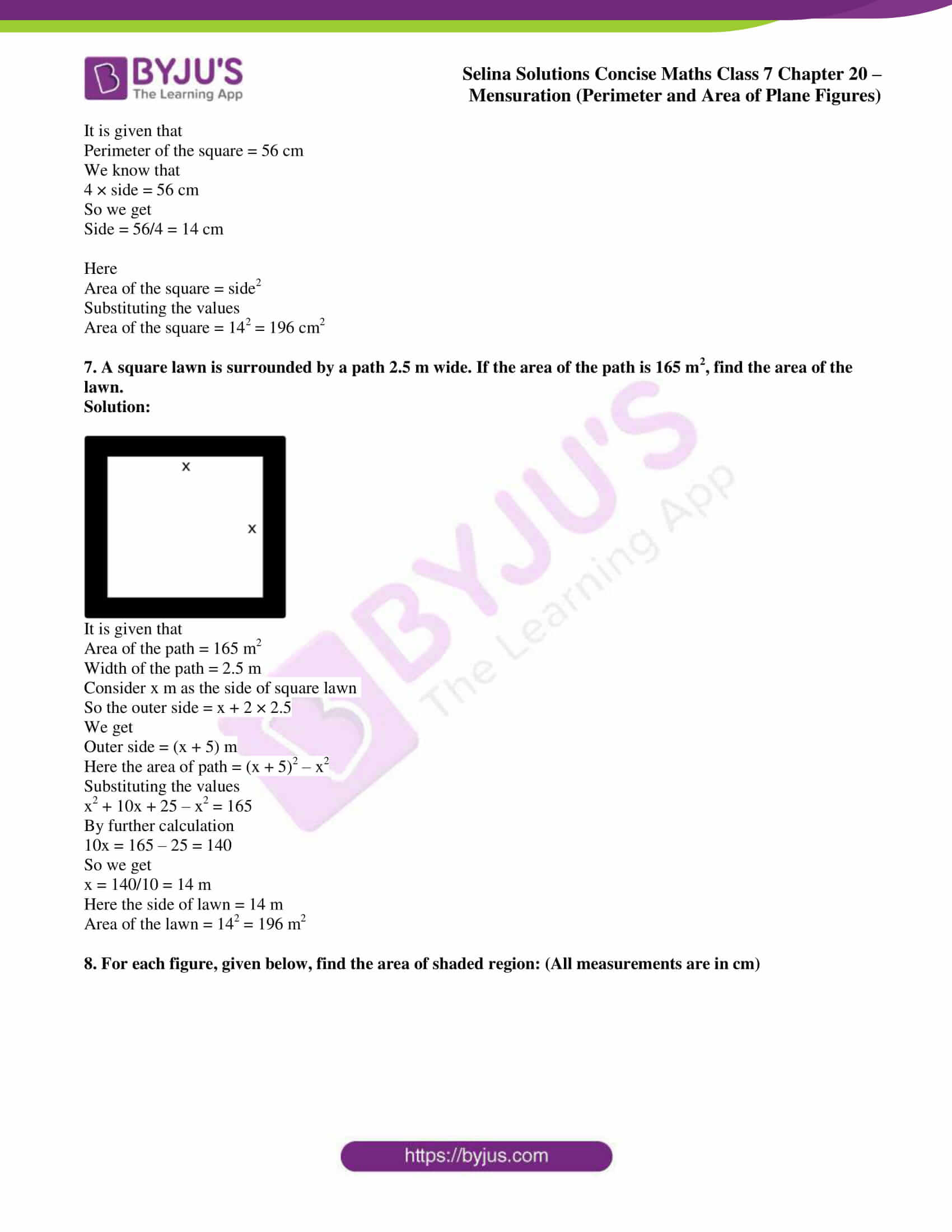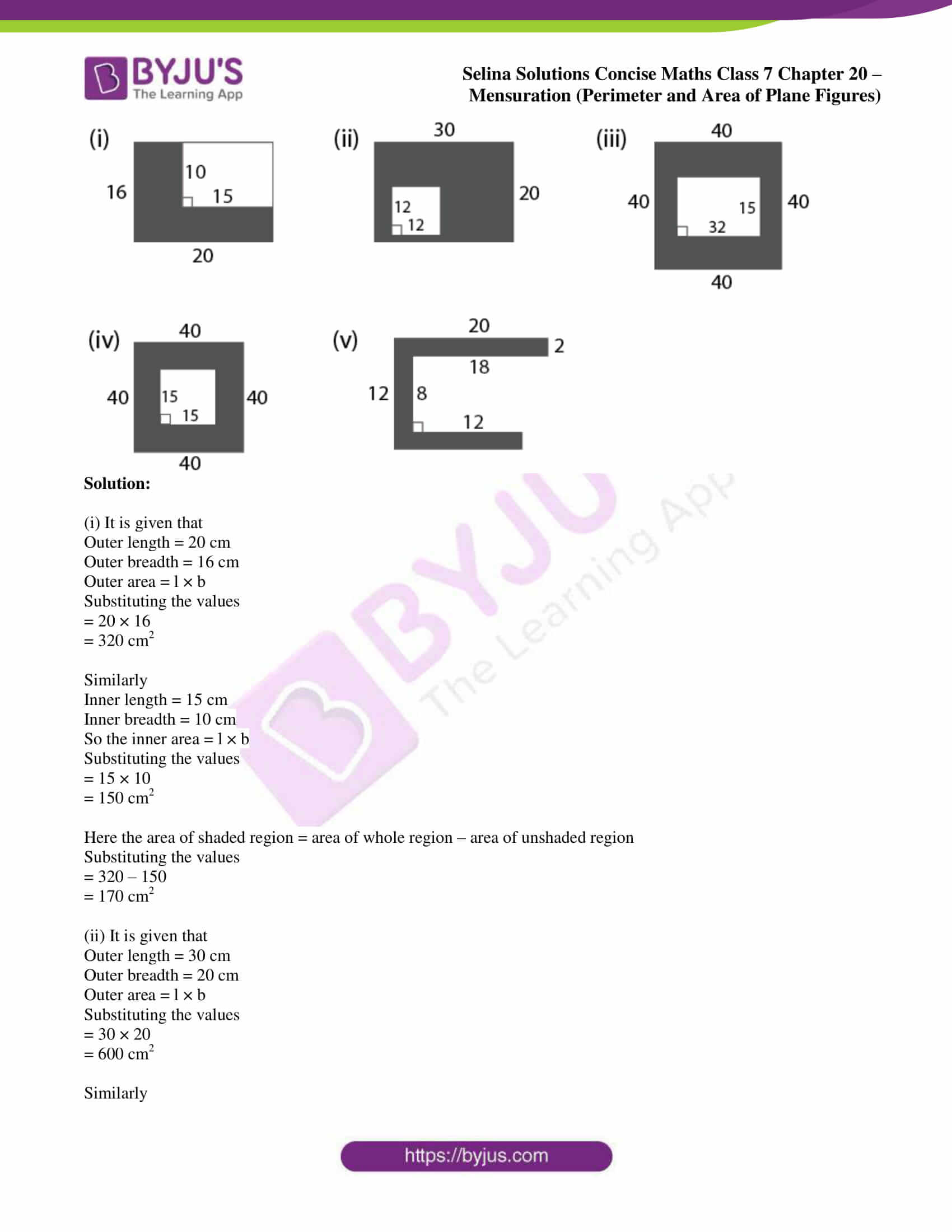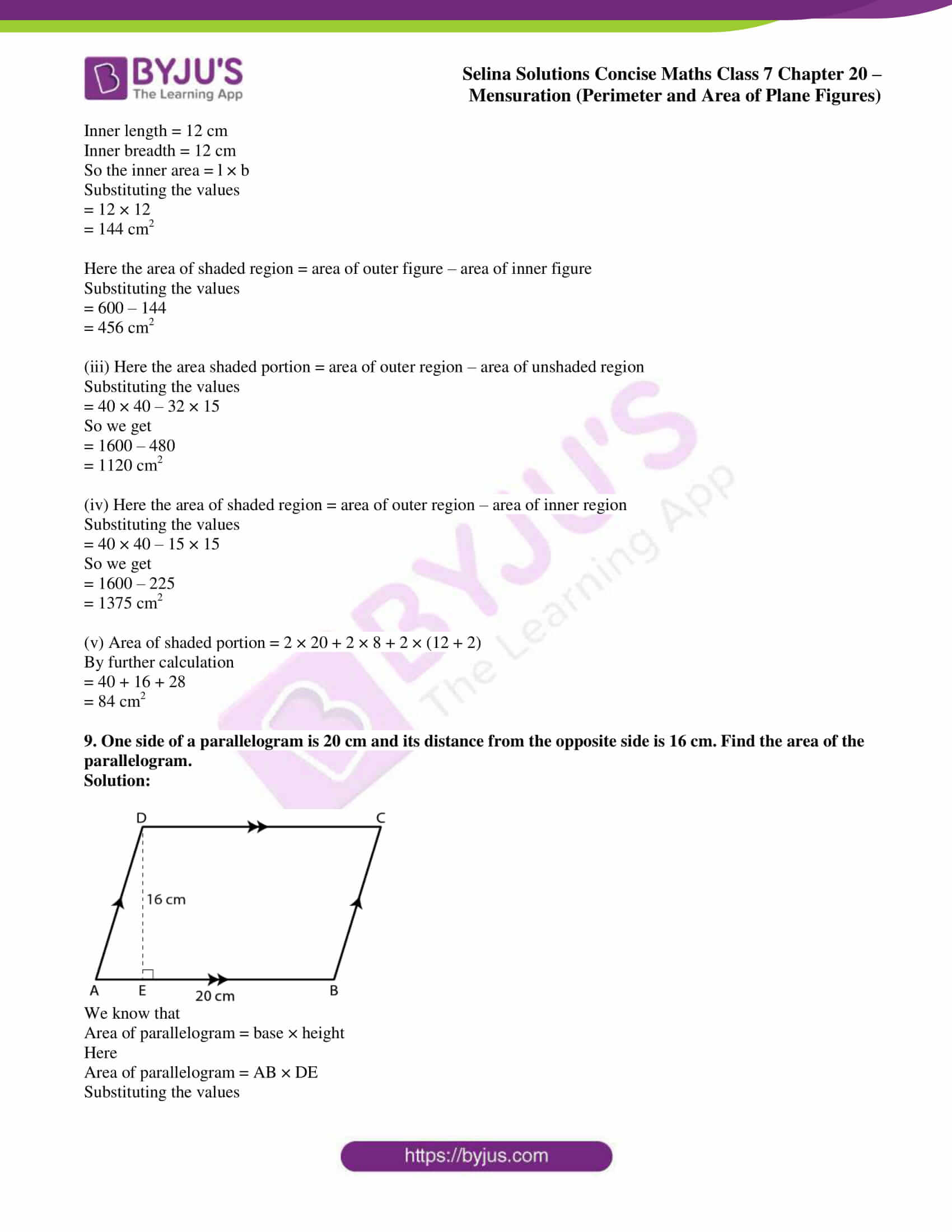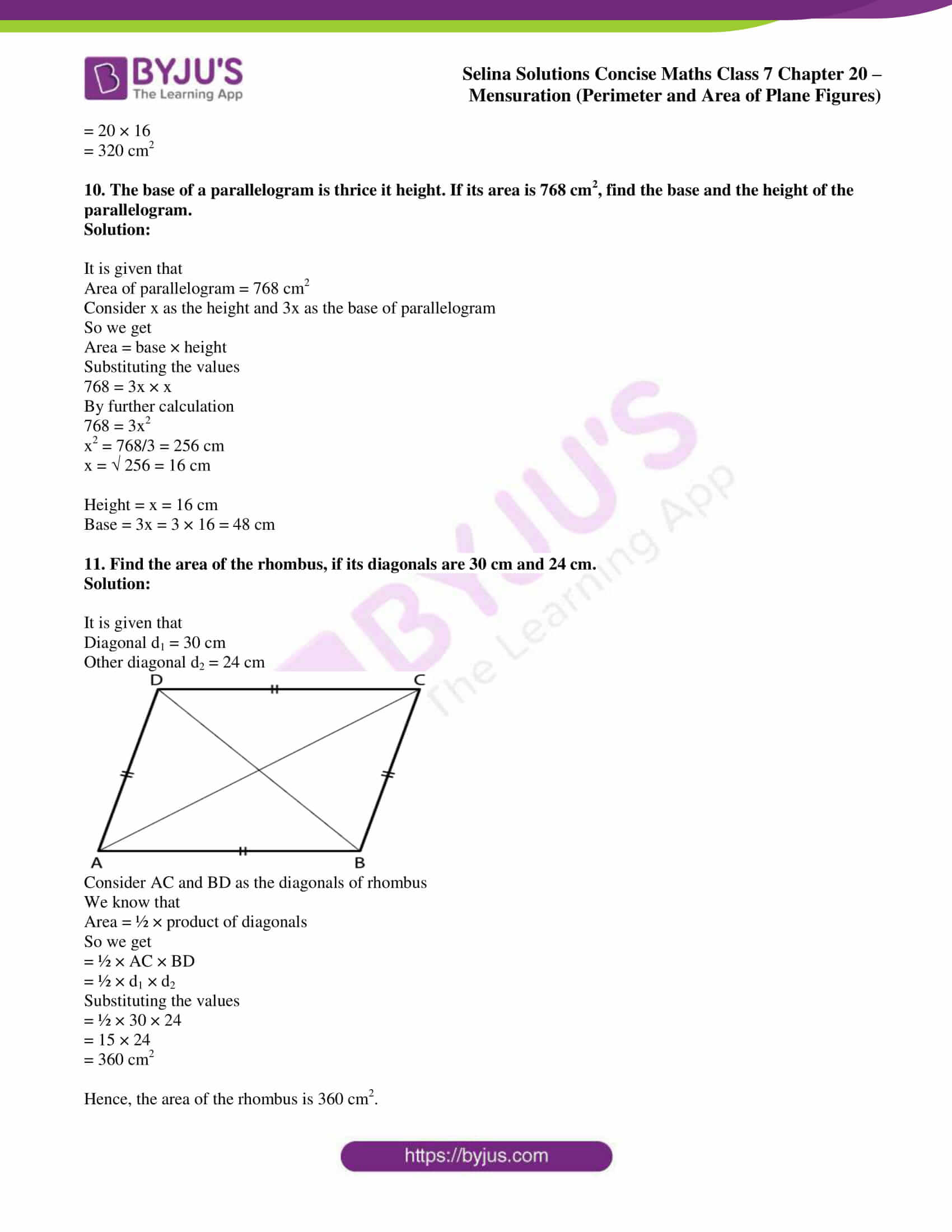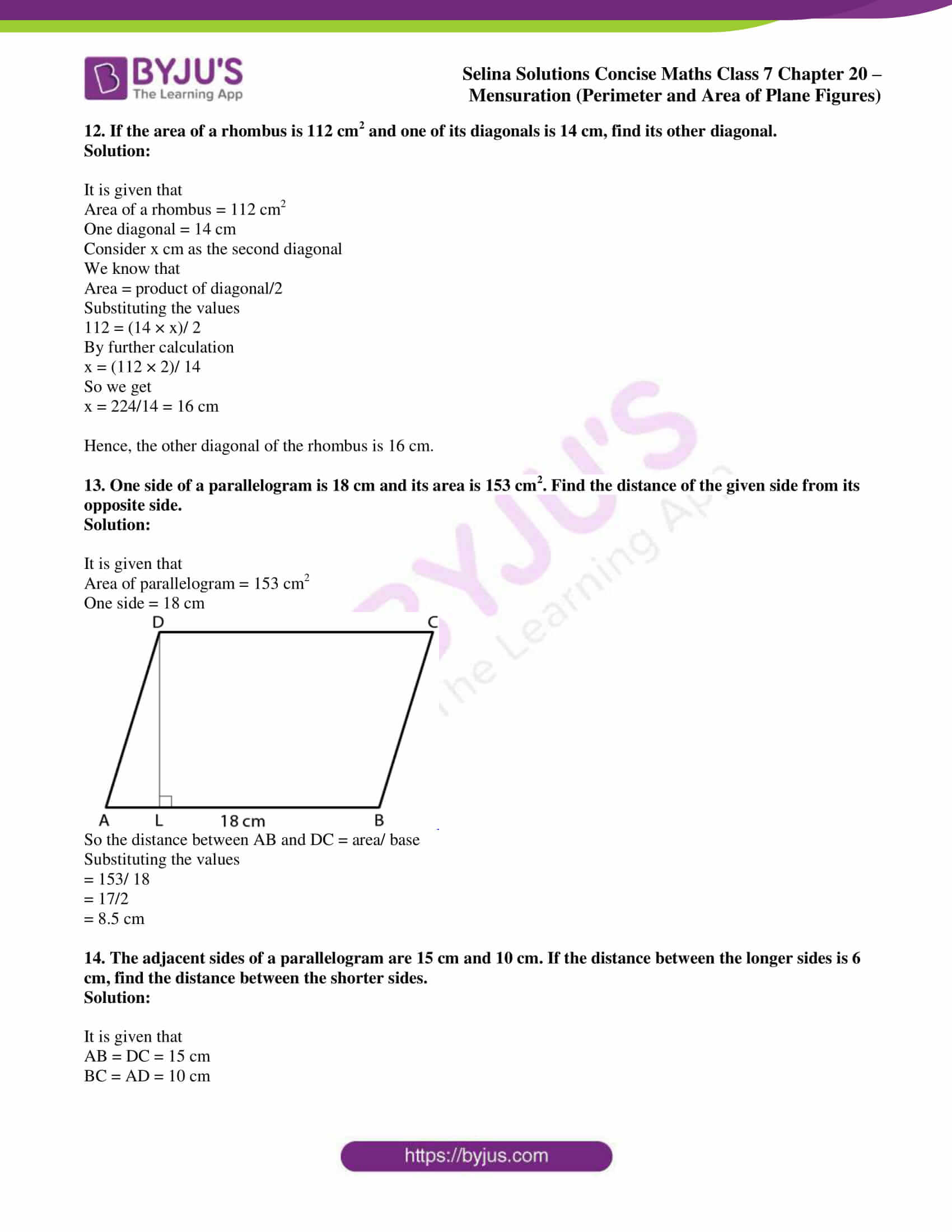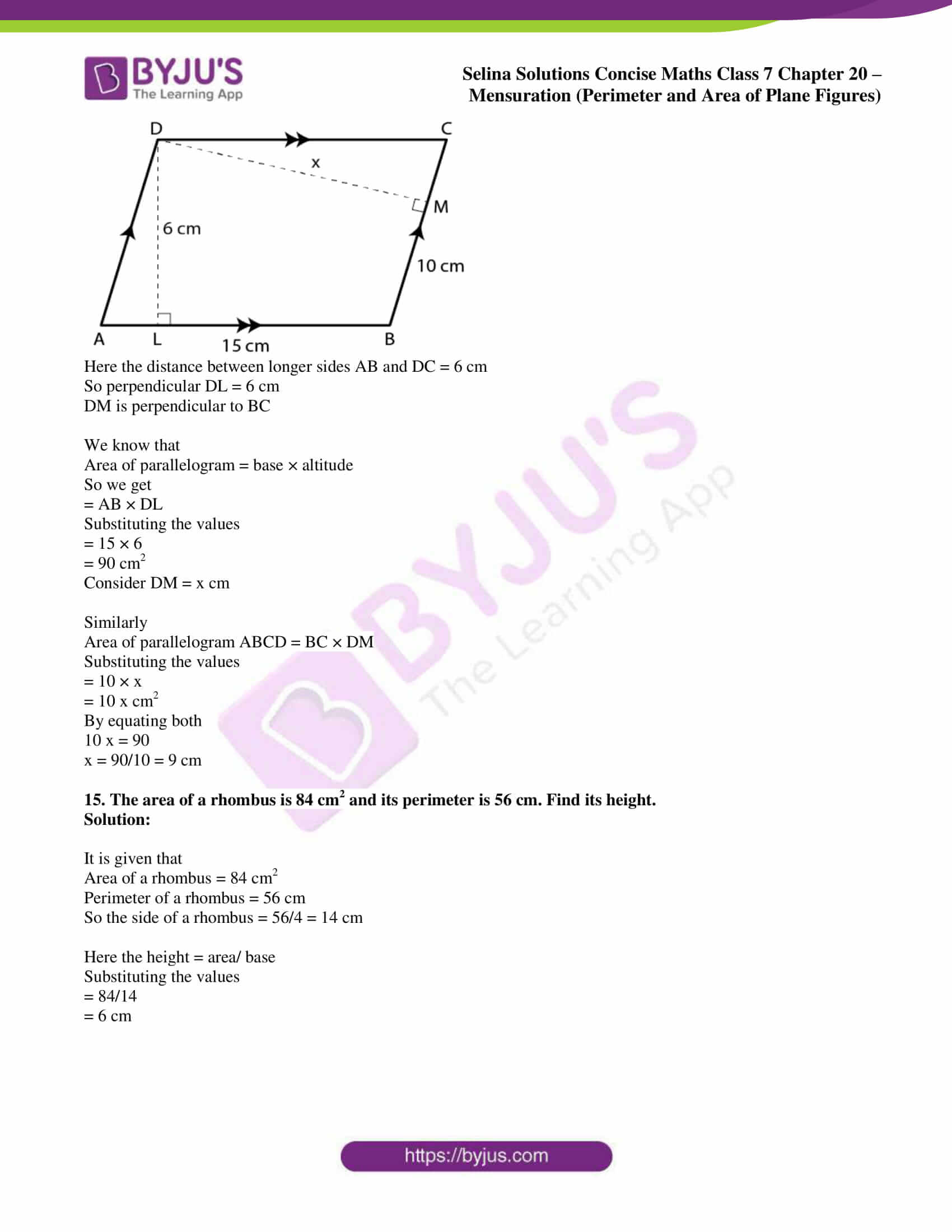### Access Selina Solutions Concise Maths Class 7 Chapter 20: Mensuration (Perimeter and Area of Plane Figures) Exercise 20B

#### Exercise 20B page: 228

1. Find the area of a rectangle whose length and breadth are 25 cm and 16 cm.

Solution:

It is given that

Length of a rectangle = 25 cm

Breadth of a rectangle = 16 cm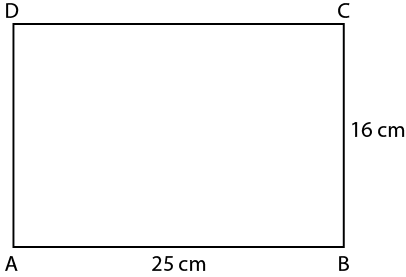We know that

Area of a rectangle = l × b

Substituting the values

= 25 × 16

= 400 cm2

2. The diagonal of a rectangular board is 1 m and its length is 96 cm. Find the area of the board.

Solution:

It is given that

Length of rectangular board = 96 cm

Diagonal of rectangular board = 1 m = 100 cm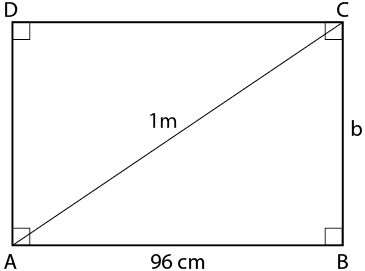Using the Pythagoras theorem in right angled triangle ABC

AC2 = AB2 + BC2

Substituting the values

1002 = 962 + BC2

By further calculation

10000 = 9216 + BC2

So we get

BC2 = 10000 – 9216 = 784

BC = 784 = 28 cm

Here the area of rectangular board = l × b

Substituting the values

= 96 × 28

= 2688 cm2

3. The sides of a rectangular park are in the ratio 4 : 3. If its area is 1728 m2, find:

(i) its perimeter

(ii) cost of fencing it at the rate of ₹40 per meter.

Solution:

It is given that

Ratio in the sides of a rectangular park = 4 : 3

Area = 1728 m2

Consider 4x as the length and 3x as the breadth

We know that

Area = l × b

Substituting the values

1728 = 4x × 3x

By further calculation

12x2 = 1728

x2= 1728/12 = 144

x = √144 = 12

Here we get

Length = 4x = 4 × 12 = 48 m

Breadth = 3x = 3 × 12 = 36 m

(i) We know that

Perimeter = 2 (l + b)

Substituting the values

= 2 (48 + 36)

So we get

= 2 (84)

= 168 m

(ii) It is given that

Rate of fencing = ₹40 per meter

So the total cost of fencing = 168 × 40 = ₹ 6720

4. A floor is 40 m long and 15 m broad. It is covered with tiles, each measuring 60 cm by 50 cm. Find the number of tiles required to cover the floor.

Solution:

Given below are the dimensions of floor

Length = 40 m

We know that

Area = l × b = 40 × 15 = 600 m2

Here the length of one tile = 60 cm = 6/10 m

Breadth of one tile = 50 cm = 5/10 m

So the area of one tile = 6/10 × 5/10 = 30/100 = 3/10 m2

We know that

Number of tiles = total area of floor/ area of one tile

Substituting the values

= 600/ 3/10

= (600 × 10)/ 3

= 2000

5. The length and breadth of a rectangular piece of land are in the ratio 5 : 3. If the total cost of fencing it at the rate of ₹24 per meter is ₹9600, find its:

(ii) area

(iii) cost of levelling at the rate of ₹60 per m2.

Solution:

It is given that

Ratio in length and breadth of a rectangular piece of land = 5 : 3

Cost of fencing = ₹9600

Rate = ₹24 per meter

We know that

Perimeter = total cost of fencing/ rate per meter

Substituting the values

= 9600 /24

= 400 m

Consider 5x as the length and 3x as the breadth

Here perimeter = 2 (l + b)

Substituting the values

400 = 2 (5x + 3x)

By further calculation

400 = 2 (8x)

So we get

400 = 16x

x = 400/16 = 25

(i) Length of land = 5x = 5 × 25 = 125 m

Breadth of land = 3x = 3 × 25 = 75 m

(ii) Area = l × b

Substituting the values

= 125 × 75

= 9375 m2

(iii) Cost of levelling at the rate of ₹60 per m2 = 60 × 9375 = ₹ 5,62,500

6. Find the area of the square whose perimeter is 56 cm.

Solution:

It is given that

Perimeter of the square = 56 cm

We know that

4 × side = 56 cm

So we get

Side = 56/4 = 14 cm

Here

Area of the square = side2

Substituting the values

Area of the square = 142 = 196 cm2

7. A square lawn is surrounded by a path 2.5 m wide. If the area of the path is 165 m2, find the area of the lawn.

Solution: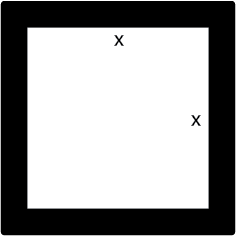It is given that

Area of the path = 165 m2

Width of the path = 2.5 m

Consider x m as the side of square lawn

So the outer side = x + 2 × 2.5

We get

Outer side = (x + 5) m

Here the area of path = (x + 5)2 – x2

Substituting the values

x2 + 10x + 25 – x2 = 165

By further calculation

10x = 165 – 25 = 140

So we get

x = 140/10 = 14 m

Here the side of lawn = 14 m

Area of the lawn = 142 = 196 m2

8. For each figure, given below, find the area of shaded region: (All measurements are in cm)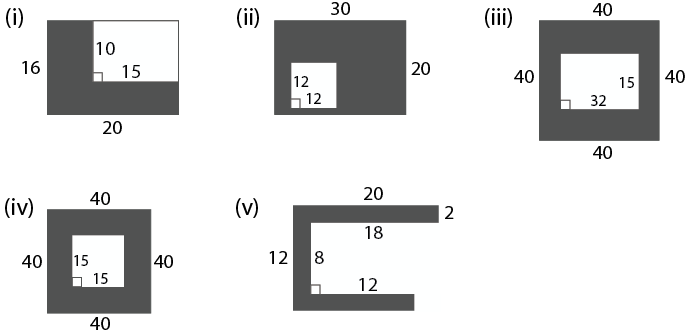Solution:

(i) It is given that

Outer length = 20 cm

Outer area = l × b

Substituting the values

= 20 × 16

= 320 cm2

Similarly

Inner length = 15 cm

So the inner area = l × b

Substituting the values

= 15 × 10

= 150 cm2

Here the area of shaded region = area of whole region – area of unshaded region

Substituting the values

= 320 – 150

= 170 cm2

(ii) It is given that

Outer length = 30 cm

Outer area = l × b

Substituting the values

= 30 × 20

= 600 cm2

Similarly

Inner length = 12 cm

So the inner area = l × b

Substituting the values

= 12 × 12

= 144 cm2

Here the area of shaded region = area of outer figure – area of inner figure

Substituting the values

= 600 – 144

= 456 cm2

(iii) Here the area shaded portion = area of outer region – area of unshaded region

Substituting the values

= 40 × 40 – 32 × 15

So we get

= 1600 – 480

= 1120 cm2

(iv) Here the area of shaded region = area of outer region – area of inner region

Substituting the values

= 40 × 40 – 15 × 15

So we get

= 1600 – 225

= 1375 cm2

(v) Area of shaded portion = 2 × 20 + 2 × 8 + 2 × (12 + 2)

By further calculation

= 40 + 16 + 28

= 84 cm2

9. One side of a parallelogram is 20 cm and its distance from the opposite side is 16 cm. Find the area of the parallelogram.

Solution: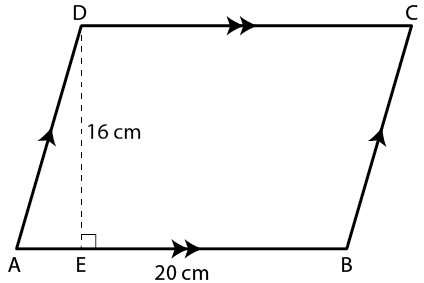We know that

Area of parallelogram = base × height

Here

Area of parallelogram = AB × DE

Substituting the values

= 20 × 16

= 320 cm2

10. The base of a parallelogram is thrice it height. If its area is 768 cm2, find the base and the height of the parallelogram.

Solution:

It is given that

Area of parallelogram = 768 cm2

Consider x as the height and 3x as the base of parallelogram

So we get

Area = base × height

Substituting the values

768 = 3x × x

By further calculation

768 = 3x2

x2 = 768/3 = 256 cm

x = √ 256 = 16 cm

Height = x = 16 cm

Base = 3x = 3 × 16 = 48 cm

11. Find the area of the rhombus, if its diagonals are 30 cm and 24 cm.

Solution:

It is given that

Diagonal d1 = 30 cm

Other diagonal d2 = 24 cm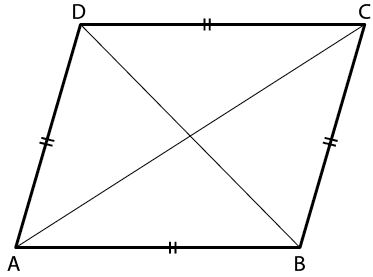Consider AC and BD as the diagonals of rhombus

We know that

Area = ½ × product of diagonals

So we get

= ½ × AC × BD

= ½ × d1 × d2

Substituting the values

= ½ × 30 × 24

= 15 × 24

= 360 cm2

Hence, the area of the rhombus is 360 cm2.

12. If the area of a rhombus is 112 cm2 and one of its diagonals is 14 cm, find its other diagonal.

Solution:

It is given that

Area of a rhombus = 112 cm2

One diagonal = 14 cm

Consider x cm as the second diagonal

We know that

Area = product of diagonal/2

Substituting the values

112 = (14 × x)/ 2

By further calculation

x = (112 × 2)/ 14

So we get

x = 224/14 = 16 cm

Hence, the other diagonal of the rhombus is 16 cm.

13. One side of a parallelogram is 18 cm and its area is 153 cm2. Find the distance of the given side from its opposite side.

Solution:

It is given that

Area of parallelogram = 153 cm2

One side = 18 cm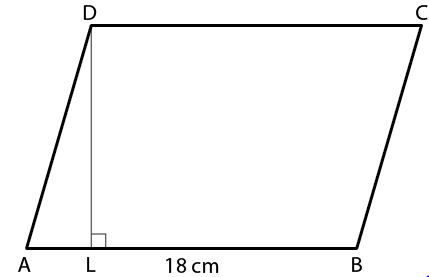So the distance between AB and DC = area/ base

Substituting the values

= 153/ 18

= 17/2

= 8.5 cm

14. The adjacent sides of a parallelogram are 15 cm and 10 cm. If the distance between the longer sides is 6 cm, find the distance between the shorter sides.

Solution:

It is given that

AB = DC = 15 cm

BC = AD = 10 cm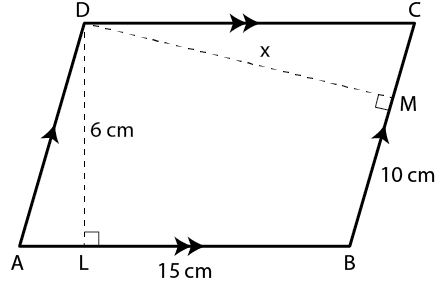Here the distance between longer sides AB and DC = 6 cm

So perpendicular DL = 6 cm

DM is perpendicular to BC

We know that

Area of parallelogram = base × altitude

So we get

= AB × DL

Substituting the values

= 15 × 6

= 90 cm2

Consider DM = x cm

Similarly

Area of parallelogram ABCD = BC × DM

Substituting the values

= 10 × x

= 10 x cm2

By equating both

10 x = 90

x = 90/10 = 9 cm

15. The area of a rhombus is 84 cm2 and its perimeter is 56 cm. Find its height.

Solution:

It is given that

Area of a rhombus = 84 cm2

Perimeter of a rhombus = 56 cm

So the side of a rhombus = 56/4 = 14 cm

Here the height = area/ base

Substituting the values

= 84/14

= 6 cm